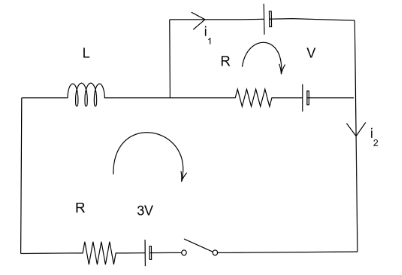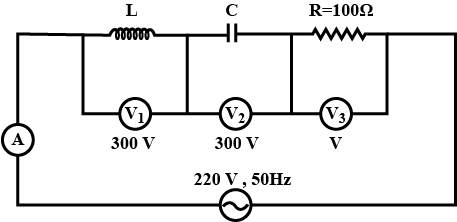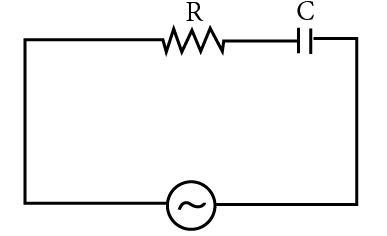Filters
Latest Questions
Physics
Ac circuits
How do you find the current in the LC circuit?
Physics
Ac circuits
Is ${\text{V}}$ the reference vector for parallel ${\text{RC}}$ circuits?
Physics
Ac circuits
An L-C circuit consists of an inductor with $L = 0.0900H$ and a capacitor of $C = 4 \times {10^{ - 4}}F$ . The initial charge on the capacitor is $5.00\mu C$ , and the initial current in the inductor is zero. When the current in the inductor has half its maximum value, what is the charge on the capacitor?
$(A)1.33 \times {10^6}C$
$(B)9.33 \times {10^{ - 6}}C$
$(C)4.33 \times {10^{ - 6}}C$
$(D)7.33 \times {10^6}C$

Physics
Ac circuits
In the LR circuit shown, what is the variation of the current $I$ as a function of time? The switch is closed at time $t = 0s$Physics
Ac circuits
Assertion (A): In a series R-L-C circuit the voltage across resistor, inductor and capacitor are $8V,16V$ and $10V$ respectively. The resultant emf in the circuit is $10V$ .
Reason (R): Resultant emf of the circuit is given by the relation, $E = \sqrt {V_R^2 + {{({V_L} - {V_C})}^2}}$ .
A. Both assertion and reason are correct and reason is the correct explanation for assertion.
B. Both assertion and reason are correct but reason is not the correct explanation for the answer.
C. Assertion is correct but reason is not correct.
D. Both assertion and reason are incorrect.
Physics
Ac circuits
In an $LCR$ circuit, capacitance is changed from $C$ to $2C$. For the resonant frequency to remain unchanged, the inductance should be changed from $L$ to :
A. $4L$
B. $\dfrac{L}{2}$
C. $2L$
D. $\dfrac{L}{4}$
Physics
Ac circuits
Voltage and current in an ac circuit are given by $V = 5\sin \left( {100\pi t - \dfrac{\pi }{6}} \right)$ and $I = 4\sin \left( {100\pi t + \dfrac{\pi }{6}} \right)$.
A. Voltage leads the current by $30^\circ$
B. Current leads the voltage by $60^\circ$
C. Voltage leads the current by $60^\circ$
D. Current and voltage are in phase
Physics
Ac circuits
In the circuit shown below, what will be the readings of the voltmeter ${V_3}$ ​and ammeter $A$ ?$(A)800V,2A$
$(B)200V,2A$
$(C)220V,2.2A$
$(D)100V,2V$

Physics
Ac circuits
A $50Hz$ AC source of $20V$ is connected across R and C as shown in figure below. The voltage across R is $12V$ . The voltage across C is
A) $8V$
B) $16V$
C) $10V$
D) Not possible to determine unless values of R and C are given.Physics
Ac circuits
What is the frequency of power in the a.c circuit when connected to a capacitor? Given $f = 50{\text{ Hz}}$ and $V = 220{\text{ V}}$.
A. ${\text{ 50 Hz}}$
B. ${\text{ 100 Hz}}$
C. ${\text{ 60 Hz}}$
D. Zero ${\text{Hz}}$
Physics
Ac circuits
A resistance of $300\Omega$ and an inductance of $\dfrac{1}{\pi }$ henry are connected in series to an A.C. voltage of $20 volts$ and $200Hz$ frequency. The phase angle between the voltage and current is:-
A. ${\tan ^{ - 1}}\left( {\dfrac{4}{3}} \right)$
B. ${\tan ^{ - 1}}\left( {\dfrac{3}{4}} \right)$
C. ${\tan ^{ - 1}}\left( {\dfrac{3}{2}} \right)$
D. ${\tan ^{ - 1}}\left( {\dfrac{2}{3}} \right)$
Physics
Ac circuits
Energy dissipated in LCR circuit in :
A. L only
B. C only
C. R only
D. All of these
Prev
1
2
3
4
5